Next: 2.2.2 Equivalence to a Up: 2.2 Mapping to a Previous: 2.2 Mapping to a

## 2.2.1 Principles of the flow-equation method

The general framework of the flow equation method(14) and its application to the Kondo model has been explained in detail in Ref. (16). Here we will only repeat the main steps in order to make this chapter self-contained, and refer to Ref. (16) for more details.

The key idea of the flow equation approach consists in performing a continuous sequence of infinitesimal unitary transformations on a given Hamiltonian(2.2)

With an anti-Hermitian generatorthe solution of equation (2.2) describes a family of unitarily equivalent Hamiltonians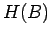parameterized by the flow parameter. By choosingappropriately(14) one can set up a framework that diagonalizes a many-particle Hamiltonian, i.e.becomes diagonal.

The concrete realization of this approach for the Kondo model was discussed in Ref. (16). The starting point is the bosonized form(17) of the Hamiltonian (2.1). Since we will be mainly interested in describing the basic ideas of our approach, we restrict ourselves to a linear dispersion relation. Notice, however, that the flow equation approach can also be used for a nontrivial conduction band density of states as it does not rely on the integrability of the model.(18) With a linear dispersion relation the Kondo problem becomes effectively one-dimensional, the charge density excitations in (2.1) decouple, and we only need to look at the spin density part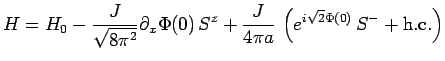(2.3)

with. Hereare the bosonic spin density modes with the bosonic spin density field defined by. For simplicity we have set the Fermi velocity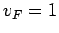.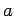is proportional to the inverse conduction band width. All our latter results will be expressed as universal functions of the low-energy Kondo scale, and we can consider (2.3) to be equivalent to our original Kondo Hamiltonian if.

Eq. (2.3) was used as the starting pointof the flow equation approach in Ref. (16). Away from the Toulouse point the unitary equivalence of the flow holds only approximately, but this approximation can be controlled by a small parameter(15) and yields very accurate results. During the flow the Hamiltonian can be parameterized as(2.4)Here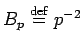, anddenote normalized vertex operators with scaling dimensionin momentum space,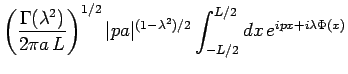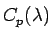that obeyand. For the special case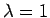they fulfill fermionic anticommutation relationsand can therefore be interpreted as creation and annihilation operators for fermions.

In Ref. (16) the following flow equations for the parameters in (2.4) have been derived(2.5)(2.6)

and a differential equation for the flow of the scaling dimension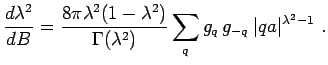(2.7)

It can be shown(16) that one always finds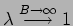in the strong-coupling phase of the Kondo model, i.e. in the low-energy limit the vertex operators in (2.4) become fermions. In the following we will use an improved version of the above flow equations by taking into account that all approximations should be performed with respect to the interacting ground state: It turns out that the only necessary modification in (2.5), (2.6) and (2.7) is that the exponent in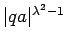gets replaced by, i.e. it is not a running exponent anymore.(19)Next: 2.2.2 Equivalence to a Up: 2.2 Mapping to a Previous: 2.2 Mapping to a
© Cyrill Slezak